• 机器学习实战第三章决策树代码，说实话感觉这一章不太实用
• ## 机器学习 --- 决策树python

千次阅读 多人点赞 2020-10-22 20:58:16
本实训项目的主要内容是基于 python 语言搭建出决策树模型对数据分类，并使用 sklearn 的决策时模型对鸢尾花数据进行分类。 信息熵与信息增益 import numpy as np def calcInfoGain(feature, label, index): ''' ...
简介
决策树说通俗点就是一棵能够替我们做决策的树，或者说是我们人类在要做决策时脑回路的一种表现形式。
本实训项目的主要内容是基于 python 语言搭建出决策树模型对数据分类，并使用 sklearn 的决策时模型对鸢尾花数据进行分类。
信息熵与信息增益
import numpy as np

def calcInfoGain(feature, label, index):
'''
计算信息增益
:param feature:测试用例中字典里的feature，类型为ndarray
:param label:测试用例中字典里的label，类型为ndarray
:param index:测试用例中字典里的index，即feature部分特征列的索引。该索引指的是feature中第几个特征，如index:0表示使用第一个特征来计算信息增益。
:return:信息增益，类型float
'''

#*********** Begin ***********#

# 计算熵
def calcInfoEntropy(feature, label):
'''
计算信息熵
:param feature:数据集中的特征，类型为ndarray
:param label:数据集中的标签，类型为ndarray
:return:信息熵，类型float
'''

label_set = set(label)
result = 0
for l in label_set:
count = 0
for j in range(len(label)):
if label[j] == l:
count += 1
# 计算标签在数据集中出现的概率
p = count / len(label)
# 计算熵
result -= p * np.log2(p)
return result

# 计算条件熵
def calcHDA(feature, label, index, value):
'''
计算信息熵
:param feature:数据集中的特征，类型为ndarray
:param label:数据集中的标签，类型为ndarray
:param index:需要使用的特征列索引，类型为int
:param value:index所表示的特征列中需要考察的特征值，类型为int
:return:信息熵，类型float
'''
count = 0
# sub_feature和sub_label表示根据特征列和特征值分割出的子数据集中的特征和标签
sub_feature = []
sub_label = []
for i in range(len(feature)):
if feature[i][index] == value:
count += 1
sub_feature.append(feature[i])
sub_label.append(label[i])
pHA = count / len(feature)
e = calcInfoEntropy(sub_feature, sub_label)
return pHA * e

base_e = calcInfoEntropy(feature, label)
f = np.array(feature)
# 得到指定特征列的值的集合
f_set = set(f[:, index])
sum_HDA = 0
# 计算条件熵
for value in f_set:
sum_HDA += calcHDA(feature, label, index, value)
# 计算信息增益
return base_e - sum_HDA
#*********** End *************#

使用ID3算法构建决策树
import numpy as np

class DecisionTree(object):
def __init__(self):
#决策树模型
self.tree = {}

def calcInfoGain(self, feature, label, index):
'''
计算信息增益
:param feature:测试用例中字典里的feature，类型为ndarray
:param label:测试用例中字典里的label，类型为ndarray
:param index:测试用例中字典里的index，即feature部分特征列的索引。该索引指的是feature中第几个特征，如index:0表示使用第一个特征来计算信息增益。
:return:信息增益，类型float
'''

# 计算熵
def calcInfoEntropy(label):
'''
计算信息熵
:param label:数据集中的标签，类型为ndarray
:return:信息熵，类型float
'''

label_set = set(label)
result = 0
for l in label_set:
count = 0
for j in range(len(label)):
if label[j] == l:
count += 1
# 计算标签在数据集中出现的概率
p = count / len(label)
# 计算熵
result -= p * np.log2(p)
return result

# 计算条件熵
def calcHDA(feature, label, index, value):
'''
计算信息熵
:param feature:数据集中的特征，类型为ndarray
:param label:数据集中的标签，类型为ndarray
:param index:需要使用的特征列索引，类型为int
:param value:index所表示的特征列中需要考察的特征值，类型为int
:return:信息熵，类型float
'''
count = 0
# sub_feature和sub_label表示根据特征列和特征值分割出的子数据集中的特征和标签
sub_feature = []
sub_label = []
for i in range(len(feature)):
if feature[i][index] == value:
count += 1
sub_feature.append(feature[i])
sub_label.append(label[i])
pHA = count / len(feature)
e = calcInfoEntropy(sub_label)
return pHA * e

base_e = calcInfoEntropy(label)
f = np.array(feature)
# 得到指定特征列的值的集合
f_set = set(f[:, index])
sum_HDA = 0
# 计算条件熵
for value in f_set:
sum_HDA += calcHDA(feature, label, index, value)
# 计算信息增益
return base_e - sum_HDA

# 获得信息增益最高的特征
def getBestFeature(self, feature, label):
max_infogain = 0
best_feature = 0
for i in range(len(feature)):
infogain = self.calcInfoGain(feature, label, i)
if infogain > max_infogain:
max_infogain = infogain
best_feature = i
return best_feature

def createTree(self, feature, label):
# 样本里都是同一个label没必要继续分叉了
if len(set(label)) == 1:
return label
# 样本中只有一个特征或者所有样本的特征都一样的话就看哪个label的票数高
if len(feature) == 1 or len(np.unique(feature, axis=0)) == 1:
vote = {}
for l in label:
if l in vote.keys():
vote[l] += 1
else:
vote[l] = 1
max_count = 0
vote_label = None
for k, v in vote.items():
if v > max_count:
max_count = v
vote_label = k
return vote_label

# 根据信息增益拿到特征的索引
best_feature = self.getBestFeature(feature, label)
tree = {best_feature: {}}
f = np.array(feature)
# 拿到bestfeature的所有特征值
f_set = set(f[:, best_feature])
# 构建对应特征值的子样本集sub_feature, sub_label
for v in f_set:
sub_feature = []
sub_label = []
for i in range(len(feature)):
if feature[i][best_feature] == v:
sub_feature.append(feature[i])
sub_label.append(label[i])
# 递归构建决策树
tree[best_feature][v] = self.createTree(sub_feature, sub_label)
return tree

def fit(self, feature, label):
'''
:param feature: 训练集数据，类型为ndarray
:param label:训练集标签，类型为ndarray
:return: None
'''

#************* Begin ************#
self.tree = self.createTree(feature, label)
#************* End **************#

def predict(self, feature):
'''
:param feature:测试集数据，类型为ndarray
:return:预测结果，如np.array([0, 1, 2, 2, 1, 0])
'''

#************* Begin ************#
result = []

def classify(tree, feature):
if not isinstance(tree, dict):
return tree
t_index, t_value = list(tree.items())
f_value = feature[t_index]
if isinstance(t_value, dict):
classLabel = classify(tree[t_index][f_value], feature)
return classLabel
else:
return t_value

for f in feature:
result.append(classify(self.tree, f))

return np.array(result)
#************* End **************#

信息增益率
import numpy as np

def calcInfoGain(feature, label, index):
'''
计算信息增益
:param feature:测试用例中字典里的feature，类型为ndarray
:param label:测试用例中字典里的label，类型为ndarray
:param index:测试用例中字典里的index，即feature部分特征列的索引。该索引指的是feature中第几个特征，如index:0表示使用第一个特征来计算信息增益。
:return:信息增益，类型float
'''
# 计算熵
def calcInfoEntropy(label):
'''
计算信息熵
:param label:数据集中的标签，类型为ndarray
:return:信息熵，类型float
'''

label_set = set(label)
result = 0
for l in label_set:
count = 0
for j in range(len(label)):
if label[j] == l:
count += 1
# 计算标签在数据集中出现的概率
p = count / len(label)
# 计算熵
result -= p * np.log2(p)
return result

# 计算条件熵
def calcHDA(feature, label, index, value):
'''
计算信息熵
:param feature:数据集中的特征，类型为ndarray
:param label:数据集中的标签，类型为ndarray
:param index:需要使用的特征列索引，类型为int
:param value:index所表示的特征列中需要考察的特征值，类型为int
:return:信息熵，类型float
'''
count = 0
# sub_feature和sub_label表示根据特征列和特征值分割出的子数据集中的特征和标签
sub_feature = []
sub_label = []
for i in range(len(feature)):
if feature[i][index] == value:
count += 1
sub_feature.append(feature[i])
sub_label.append(label[i])
pHA = count / len(feature)
e = calcInfoEntropy(sub_label)
return pHA * e

base_e = calcInfoEntropy(label)
f = np.array(feature)
# 得到指定特征列的值的集合
f_set = set(f[:, index])
sum_HDA = 0
# 计算条件熵
for value in f_set:
sum_HDA += calcHDA(feature, label, index, value)
# 计算信息增益
return base_e - sum_HDA

def calcInfoGainRatio(feature, label, index):
'''
计算信息增益率
:param feature:测试用例中字典里的feature，类型为ndarray
:param label:测试用例中字典里的label，类型为ndarray
:param index:测试用例中字典里的index，即feature部分特征列的索引。该索引指的是feature中第几个特征，如index:0表示使用第一个特征来计算信息增益。
:return:信息增益率，类型float
'''

#********* Begin *********#
info_gain = calcInfoGain(feature, label, index)
unique_value = list(set(feature[:, index]))
IV = 0
for value in unique_value:
len_v = np.sum(feature[:, index] == value)
IV -= (len_v/len(feature))*np.log2((len_v/len(feature)))
return info_gain/IV
#********* End *********#

基尼系数
import numpy as np

def calcGini(feature, label, index):
'''
计算基尼系数
:param feature:测试用例中字典里的feature，类型为ndarray
:param label:测试用例中字典里的label，类型为ndarray
:param index:测试用例中字典里的index，即feature部分特征列的索引。该索引指的是feature中第几个特征，如index:0表示使用第一个特征来计算信息增益。
:return:基尼系数，类型float
'''

#********* Begin *********#
def _gini(label):
unique_label = list(set(label))
gini = 1
for l in unique_label:
p = np.sum(label == l)/len(label)
gini -= p**2
return gini
unique_value = list(set(feature[:, index]))
gini = 0
for value in unique_value:
len_v = np.sum(feature[:, index] == value)
gini += (len_v/len(feature))*_gini(label[feature[:, index] == value])
return gini
#********* End *********#

预剪枝与后剪枝
import numpy as np
from copy import deepcopy

class DecisionTree(object):
def __init__(self):
#决策树模型
self.tree = {}

def calcInfoGain(self, feature, label, index):
'''
计算信息增益
:param feature:测试用例中字典里的feature，类型为ndarray
:param label:测试用例中字典里的label，类型为ndarray
:param index:测试用例中字典里的index，即feature部分特征列的索引。该索引指的是feature中第几个特征，如index:0表示使用第一个特征来计算信息增益。
:return:信息增益，类型float
'''

# 计算熵
def calcInfoEntropy(feature, label):
'''
计算信息熵
:param feature:数据集中的特征，类型为ndarray
:param label:数据集中的标签，类型为ndarray
:return:信息熵，类型float
'''

label_set = set(label)
result = 0
for l in label_set:
count = 0
for j in range(len(label)):
if label[j] == l:
count += 1
# 计算标签在数据集中出现的概率
p = count / len(label)
# 计算熵
result -= p * np.log2(p)
return result

# 计算条件熵
def calcHDA(feature, label, index, value):
'''
计算信息熵
:param feature:数据集中的特征，类型为ndarray
:param label:数据集中的标签，类型为ndarray
:param index:需要使用的特征列索引，类型为int
:param value:index所表示的特征列中需要考察的特征值，类型为int
:return:信息熵，类型float
'''
count = 0
# sub_feature和sub_label表示根据特征列和特征值分割出的子数据集中的特征和标签
sub_feature = []
sub_label = []
for i in range(len(feature)):
if feature[i][index] == value:
count += 1
sub_feature.append(feature[i])
sub_label.append(label[i])
pHA = count / len(feature)
e = calcInfoEntropy(sub_feature, sub_label)
return pHA * e

base_e = calcInfoEntropy(feature, label)
f = np.array(feature)
# 得到指定特征列的值的集合
f_set = set(f[:, index])
sum_HDA = 0
# 计算条件熵
for value in f_set:
sum_HDA += calcHDA(feature, label, index, value)
# 计算信息增益
return base_e - sum_HDA

# 获得信息增益最高的特征
def getBestFeature(self, feature, label):
max_infogain = 0
best_feature = 0
for i in range(len(feature)):
infogain = self.calcInfoGain(feature, label, i)
if infogain > max_infogain:
max_infogain = infogain
best_feature = i
return best_feature

# 计算验证集准确率
def calc_acc_val(self, the_tree, val_feature, val_label):
result = []

def classify(tree, feature):
if not isinstance(tree, dict):
return tree
t_index, t_value = list(tree.items())
f_value = feature[t_index]
if isinstance(t_value, dict):
classLabel = classify(tree[t_index][f_value], feature)
return classLabel
else:
return t_value

for f in val_feature:
result.append(classify(the_tree, f))

result = np.array(result)
return np.mean(result == val_label)

def createTree(self, train_feature, train_label):
# 样本里都是同一个label没必要继续分叉了
if len(set(train_label)) == 1:
return train_label
# 样本中只有一个特征或者所有样本的特征都一样的话就看哪个label的票数高
if len(train_feature) == 1 or len(np.unique(train_feature, axis=0)) == 1:
vote = {}
for l in train_label:
if l in vote.keys():
vote[l] += 1
else:
vote[l] = 1
max_count = 0
vote_label = None
for k, v in vote.items():
if v > max_count:
max_count = v
vote_label = k
return vote_label

# 根据信息增益拿到特征的索引
best_feature = self.getBestFeature(train_feature, train_label)
tree = {best_feature: {}}
f = np.array(train_feature)
# 拿到bestfeature的所有特征值
f_set = set(f[:, best_feature])
# 构建对应特征值的子样本集sub_feature, sub_label
for v in f_set:
sub_feature = []
sub_label = []
for i in range(len(train_feature)):
if train_feature[i][best_feature] == v:
sub_feature.append(train_feature[i])
sub_label.append(train_label[i])

# 递归构建决策树
tree[best_feature][v] = self.createTree(sub_feature, sub_label)

return tree

# 后剪枝
def post_cut(self, val_feature, val_label):
# 拿到非叶子节点的数量
def get_non_leaf_node_count(tree):
non_leaf_node_path = []

def dfs(tree, path, all_path):
for k in tree.keys():
if isinstance(tree[k], dict):
path.append(k)
dfs(tree[k], path, all_path)
if len(path) > 0:
path.pop()
else:
all_path.append(path[:])

dfs(tree, [], non_leaf_node_path)

unique_non_leaf_node = []
for path in non_leaf_node_path:
isFind = False
for p in unique_non_leaf_node:
if path == p:
isFind = True
break
if not isFind:
unique_non_leaf_node.append(path)
return len(unique_non_leaf_node)

# 拿到树中深度最深的从根节点到非叶子节点的路径
def get_the_most_deep_path(tree):
non_leaf_node_path = []

def dfs(tree, path, all_path):
for k in tree.keys():
if isinstance(tree[k], dict):
path.append(k)
dfs(tree[k], path, all_path)
if len(path) > 0:
path.pop()
else:
all_path.append(path[:])

dfs(tree, [], non_leaf_node_path)

max_depth = 0
result = None
for path in non_leaf_node_path:
if len(path) > max_depth:
max_depth = len(path)
result = path
return result

# 剪枝
def set_vote_label(tree, path, label):
for i in range(len(path)-1):
tree = tree[path[i]]
tree[path[len(path)-1]] = vote_label

acc_before_cut = self.calc_acc_val(self.tree, val_feature, val_label)
# 遍历所有非叶子节点
for _ in range(get_non_leaf_node_count(self.tree)):
path = get_the_most_deep_path(self.tree)

# 备份树
tree = deepcopy(self.tree)
step = deepcopy(tree)

# 跟着路径走
for k in path:
step = step[k]

# 叶子节点中票数最多的标签
vote_label = sorted(step.items(), key=lambda item: item, reverse=True)

# 在备份的树上剪枝
set_vote_label(tree, path, vote_label)

acc_after_cut = self.calc_acc_val(tree, val_feature, val_label)

# 验证集准确率高于0.9才剪枝
if acc_after_cut > acc_before_cut:
set_vote_label(self.tree, path, vote_label)
acc_before_cut = acc_after_cut

def fit(self, train_feature, train_label, val_feature, val_label):
'''
:param train_feature:训练集数据，类型为ndarray
:param train_label:训练集标签，类型为ndarray
:param val_feature:验证集数据，类型为ndarray
:param val_label:验证集标签，类型为ndarray
:return: None
'''

#************* Begin ************#
self.tree = self.createTree(train_feature, train_label)
# 后剪枝
self.post_cut(val_feature, val_label)
#************* End **************#

def predict(self, feature):
'''
:param feature:测试集数据，类型为ndarray
:return:预测结果，如np.array([0, 1, 2, 2, 1, 0])
'''

#************* Begin ************#
result = []

# 单个样本分类
def classify(tree, feature):
if not isinstance(tree, dict):
return tree
t_index, t_value = list(tree.items())
f_value = feature[t_index]
if isinstance(t_value, dict):
classLabel = classify(tree[t_index][f_value], feature)
return classLabel
else:
return t_value

for f in feature:
result.append(classify(self.tree, f))

return np.array(result)
#************* End **************#

鸢尾花识别
#********* Begin *********#
import pandas as pd
from sklearn.tree import DecisionTreeClassifier

dt = DecisionTreeClassifier()
dt.fit(train_df, train_label)
result = dt.predict(test_df)

result = pd.DataFrame({'target':result})
result.to_csv('./step7/predict.csv', index=False)

#********* End *********#

开始你的任务吧，祝你成功！！！！！！！！！！！！！


展开全文在学习机器学习决策树应用过程中，由于使用python2版本，遇到一些语法上的问题。
python2:

allElectronicsData = open(r'C:\Users\IYQ\Desktop\Electronics.csv', 'rb')

AttributeError: '_csv.reader' object has no attribute 'next'

rb报错：
_csv.Error: iterator should return strings, not bytes (did you open the file in text mode?)

Electronics.csv文件格式报错：
UnicodeDecodeError: 'gbk' codec can't decode byte 0xff in position 0: illegal multibyte sequence

python3:
allElectronicsData = open(r'C:\Users\IYQ\Desktop\Electronics.csv', 'r')


展开全文csv
• 决策树（Decision Tree）是一种基本的分类与回归方法。本文会讨论决策树中的分类树与回归树，后续文章会继续讨论决策树的Boosting和Bagging的相关方法。决策树由结点和有向边组成。结点有两种类型：内部结点和叶结点...
分类目录：《深入理解机器学习》总目录
相关文章：
基于决策树的模型（一）分类树和回归树
基于树的模型（二）：集成学习之Bagging和Random Forest
基于树的模型（三）：集成学习之GBDT和XGBoost
基于树的模型（四）：随机森林的延伸——深度森林（gcForest）
基于树的模型（五）：从零开始用Python实现ID3决策树
基于树的模型（六）：Python实现CART决策树并利用Tkinter构建GUI对决策树进行调优
基于树的模型（七）：RF/XGBoost等算法实践与决策树Scala实践等（材料准备中）

决策树（Decision Tree）是一种基本的分类与回归方法，当决策树用于分类时称为分类树，用于回归时称为回归树。本文主要讨论决策树中的分类树与回归树的一些基本理论，后续文章会继续讨论决策树的Boosting和Bagging相关方法。
决策树由结点和有向边组成。结点有两种类型：内部结点和叶结点，其中内部结点表示一个特征或属性，叶结点表示一个类。一般的，一棵决策树包含一个根结点、若干个内部结点和若干个叶结点。叶结点对应于决策结果，其他每个结点则对应于一个属性测试。每个结点包含的样本集合根据属性测试的结果被划分到子结点中，根结点包含样本全集，从根结点到每个叶结点的路径对应了一个判定测试序列。在下图中，圆和方框分别表示内部结点和叶结点。决策树学习的目的是为了产生一棵泛化能力强，即处理未见示例能力强的决策树。分类树
分类树是一种描述对实例进行分类的树形结构。在使用分类树进行分类时，从根结点开始，对实例的某一特征进行测试，根据测试结果，将实例分配到其子结点。这时，每一个子结点对应着该特征的一个取值。如此递归地对实例进行测试并分配，直至达到叶结点。最后将实例分到叶结点的类中。
假设给定训练数据集：
$D=\{(x_1, y_1), (x_2, y_2), ..., (x_N, y_N)\}$其中，$x_i=(x_i^{(1)}, x_i^{(2)}, ..., x_i^{(n)})^T,$为输入实例，即特征向量，$n$为特征个数，$i=1，2…，N$，$N$为样本容量，$y_i \in \{ 1, 2, ..., K\}$为类标。分类树学习的目标是根据给定的训练数据集构建一个决策树模型，使它能够对实例进行正确的分类。
决策树学习本质上是从训练数据集中归纳出一组分类规则。与训练数据集不相矛盾的决策树（即能对训练数据进行正确分类的决策树）可能有多个，也可能一个也没有。我们需要的是一个与训练数据矛盾较小的决策树，同时具有很好的泛化能力。从另一个角度看，决策树学习是由训练数据集估计条件概率模型。基于特征空间划分的类的条件概率模型有无穷多个，我们选择的条件概率模型应该不仅对训练数据有很好的拟合，而且对未知数据有很好的预测。
决策树学习用损失函数表示这一目标，其损失函数通常是正则化的极大似然函数，决策树学习的策略是以损失函数为目标函数的最小化。当损失函数确定以后，学习问题就变为在损失函数意义下选择最优决策树的问题。因为从所有可能的决策树中选取最优决策树是NP完全问题，所以现实中决策树学习算法通常采用启发式方法，近似求解这一最优化问题。这样得到的决策树是次最优的。

决策树分类算法
输入：
$\qquad$ 训练集：$D = {(x_1, y_1), (x_2, y_2), \cdots, (x_N, y_N)}$
$\qquad$ 属性集：$A = {a_1, a_2, \cdots, a_n}$
过程：
$\qquad$ 函数$TreeGenerate(D, A)$
输出：
$\qquad$ 以node为根节点的决策树
算法：
（ 1 ） 生成结点根node
（ 2 ） if $D$中样本全属于同一类别$C_k$ then
（ 3 ） $\quad$ 将node标记为$C_k$类叶结点
（ 4 ） $\quad$ return
（ 5 ） end if
（ 6 ） if $A = \varnothing$ OR $D$中样本在$A$上取值相同 then
（ 7 ） $\quad$ 将node标记为叶结点，其类别标记为$D$中样本数最多的类
（ 8 ） $\quad$ return
（ 9 ）end if
（10）从$A$中选择最优划分属性$a_*$
（11）for $a_*$ 的每一个值$a_*^v$ do
（12）$\quad$ 为node生成一个分支：令$D_v$表示$D$中在$a_*$上取值为$a_*^v$的样本子集
（13）$\quad$  if $D_v$为空  then
（14）$\qquad$ 将分支结点标记为叶结点，其类别标记为$D$中样本最多的类
（15）$\qquad$ return
（16）$\quad$  else
（17）$\qquad$ 以$TreeGenerate(D_v, A - \{a_*\})$为分支结点
（18）$\quad$ end if
（19）end for

决策树学习的算法通常是一个递归地选择最优特征，并根据该特征对训练数据进行分割，使得对各个子数据集有一个最好的分类的过程。这一过程对应着对特征空间的划分，也对应着决策树的构建。开始，构建根结点，将所有训练数据都放在根结点。选择一个最优特征，按照这一特征将训练数据集分割成子集，使得各个子集有一个在当前条件下最好的分类。如果这些子集已经能够被基本正确分类，那么构建叶结点，并将这些子集分到所对应的叶结点中去，如果还有子集不能被基本正确分类，那么就对这些子集选择新的最优特征，继续对其进行分割，构建相应的结点。如此递归地进行下去，直至所有训练数据子集被基本正确分类，或者没有合适的特征为止。最后每个子集都被分到叶结点上，即都有了明确的类。这就生成了一棵决策树。
从上述过程中就可以看出，决策树的生成是一个递归过程。在决策树基本算法中，有三种情形会导致递归返回

当前结点包含的样本全属于同一类别，无需划分
当前属性集为空，或是所有样本在所有属性上取值相同，无法划分
当前结点包含的样本集合为空，不能划分

在第二种情形下，我们把当前结点标记为叶结点，并将其类别设定为该结点所含样本最多的类别。在第三种情形下，同样把当前结点标记为叶结点，但将其类别设定为其父结点所含样本最多的类别。这两种情形的处理实质不同：第二种情况是在利用当前结点的后验分布，而第三种情况则是把父结点的样本分布作为当前结点的先验分布。
以上方法生成的决策树可能对训练数据有很好的分类能力，但对未知的测试数据却未必有很好的分类能力，即可能发生过拟合现象。我们需要对已生成的树自下而上进行剪枝，将树变得更简单，从而使它具有更好的泛化能力。具体地，就是去掉过于细分的叶结点，使其回退到父结点，甚至更高的结点，然后将父结点或更高的结点改为新的叶结点。如果特征数量很多，也可以在决策树学习开始的时候，对特征进行选择，只留下对训练数据有足够分类能力的特征。
可以看出，决策树学习算法包含特征选择、决策树的生成与决策树的剪枝过程。由于决策树表示一个条件概率分布，所以深浅不同的决策树对应着不同复杂度的概率模型。决策树的生成对应于模型的局部选择，决策树的剪枝对应于模型的全局选择。决策树的生成只考虑局部最优，相对地，决策树的剪枝则考虑全局最优。
决策树模型呈树形结构，在分类问题中，表示基于特征对实例进行分类的过程。它可以认为是if-then规则的集合，也可以认为是定义在特征空间与类空间上的条件概率分布。分类树具有良好的可读性与分类速度快的优点。分类树在学习时，利用训练数据，根据损失函数最小化的原则建立分类树模型，在预测时，对新的数据，利用分类树模型进行分类。决策树学习通常包括3个步骤：特征选择、决策树的生成和决策树的修剪。
决策树与if-then规则
可以将决策树看成一个if-then规则的集合：由决策树的根结点到叶结点的每一条路径构建一条规则，路径上内部结点的特征对应着规则的条件，而叶结点的类对应着规则的结论。决策树的路径或其对应的if-then规则集合具有一个重要的性质——互斥并且完备。这就是说，每一个实例都被一条路径或一条规则所覆盖，而且只被一条路径或一条规则所覆盖。这里所谓覆盖是指实例的特征与路径上的特征一致或实例满足规则的条件。
决策树与条件概率分布
决策树还表示给定特征条件下类的条件概率分布。这一条件概率分布定义在特征空间的一个划分上。将特征空间划分为互不相交的单元或区域，并在每个单元定义一个类的概率分布就构成了一个条件概率分布。决策树的一条路径对应于划分中的一个单元。决策树所表示的条件概率分布由各个单元给定条件下类的条件概率分布组成。假设$X$为表示特征的随机变量，$Y$为表示类的随机变量，那么这个条件概率分布可以表示为$P（Y|X）$。$X$取值于给定划分下单元的集合，$Y$取值于类的集合。各叶结点（单元）上的条件概率往往偏向某一个类，即属于某一类的概率较大。决策树分类时将该结点的实例强行分到条件概率大的那一类去。
决策树的优缺点

计算复杂度不高
对中间缺失值不敏感
解释性强，在解释性方面甚至比线性回归更强
与传统的回归和分类方法相比，决策树更接近人的决策模式
可以用图形表示，非专业人士也可以轻松理解
可以直接处理定性的预测变量而不需创建哑变量
决策树的预测准确性一般比回归和分类方法弱，但可以通过用集成学习方法组合大量决策树，显著提升树的预测效果

特征选择
特征选择在于选取对训练数据具有分类能力的特征。这样可以提高决策树学习的效率。如果利用一个特征进行分类的结果与随机分类的结果没有很大差别，则称这个特征是没有分类能力的。经验上扔掉这样的特征对决策树学习的精度影响不大。比如，我们希望构建一棵决策树来根据不同人的各种属性来预测每个人性别，那么对于属性“头发的长度”可能就要比属性“头发的颜色”所能包含的信息更多。因为一般来说，男生的头发要比女生的头发短，所以我们希望“头发的长度”这个属性处于决策树的上部。随着划分过程不断进行，我们希望决策树的分支结点所包含的样本尽可能属于同一类别，即结点的“纯度”（purity）越来越高。
信息增益
为了便于说明信息增益，先给出熵与条件熵的定义。在信息论与概率统计中，熵（entropy）是表示随机变量不确定性的度量。设$X$是一个取有限个值的离散随机变量，其概率分布为:
$P(X = x_i) = p_i, i = 1, 2, \cdots, n$
则随机变量$X$的熵定义为：
$H(X) = -\sum_{i = 1}^n p_i \log p_i$
在上式中，若$p_i = 0$，则定义$p_i \log p_i = 0$。通常，上式中的对数以$2$为底或以$e$为底（自然对数），这时熵的单位分别称作比特（bit）或纳特（nat）.由定义可知，熵只依赖于$X$的分布，而与$X$的取值无关，所以也可将$X$的熵记作$H(p)$，即:
$H(p) = -\sum_{i = 1}^n p_i \log p_i$
由此可见，熵越大，随机变量的不确定性就越大。从熵的定义可验证
$0 \leq H(p) \leq \log n$
当随机变量只取两个值，例如1，0时，即$X$的分布为:
$P(X = 1) = p,\quad P(X = 0) = 1-p, \quad 0≤p≤1$
其熵为：
$H(p) = -p \log_2 p - (1 - p)\log_2 (1 - p)$
这时，熵$H(p)$随概率$p$变化的曲线如下图所示（单位为比特）：当$p = 0$或$p = 1$时$H(p) = 0$，随机变量完全没有不确定，当$p = 0.5$时，$H(p) = 1$，熵取值最大，随机变量不确定性最大。
设有随机变量$(X, Y)$，其联合概率分布为：
$P(X = x_i, Y = y_i) = p_{ij} \quad \begin{cases} i = 1, 2, \cdots, n \\ j = 1, 2, \cdots, m \end{cases}$
条件熵$H(Y|X)$表示在已知随机变量$X$的条件下随机变量$Y$的不确定性。随机变量$X$给定的条件下随机变量$Y$的条件熵（conditional entropy）$H(Y|X)$，定义为$X$给定条件下$Y$的条件概率分布的熵对$X$的数学期望：
$H(Y|X) = \sum_{i = 1}^n p_iH(Y|X = x_i)$
其中，$p_i = P(X = x_i), i = 1, 2, \cdots, n$。
当熵和条件熵中的概率由数据估计（如极大似然估计）得到时，所对应的熵与条件熵分别称为经验熵（empirical entropy）和经验条件熵（empirical conditional entropy）。此时，如果有0概率，令$0\log0 = 0$。
信息增益（information gain）表示得知特征$X$的信息而使得类$Y$的信息的不确定性减少的程度。特征$a_*$对训练数据集$D$的信息增益$g(D, a_*)$，定义为集合$D$的经验熵$H(D)$与特征$a_*$给定条件下$D$的经验条件熵$H(D|a_*)$之差，即：
$g(D, a_*) = H(D) - H(D|a_*)$
一般地，熵$H(Y)$与条件熵$H(Y|X)$之差称为互信息（mutual information）。决策树学习中的信息增益等价于训练数据集中类与特征的互信息。
决策树学习应用信息增益准则选择特征。给定训练数据集$D$和特征$a_*$，经验熵$H(D)$表示对数据集$D$进行分类的不确定性。而经验条件熵$H(D|a_*)$表示在特征$a_*$给定的条件下对数据集$D$进行分类的不确定性。那么它们的差，即信息增益，就表示由于特征$a_*$而使得对数据集$D$的分类的不确定性减少的程度。显然，对于数据集$D$而言，信息增益依赖于特征，不同的特征往往具有不同的信息增益，信息增益大的特征具有更强的分类能力。
根据信息增益准则的特征选择方法：对训练数据集（或子集）$D$，计算其每个特征的信息增益，并比较它们的大小，选择信息增益最大的特征。
设训练数据集为$D$，$|D|$表示其样本容量，即样本个数。设有$K$个类$C_k$，$k=1, 2, \cdots, K$，$|C_k|$为属于类$C_k$的样本个数，$\sum_{k=1}^K |C_k| = |D|$。设特征$a_*$有$V$个不同的取值$\{ a_*^1, a_*^2, \cdots, a_*^V\}$，根据特征$a_*$的取值将$D$划分为$V$个子集$D_1, D_2, \cdots, D_V$，$|D_t|$为$D_t$的样本个数，$\sum_{i=1}^n|D_t|=|D|$。记子集$D_i$中属于类$C_k$的样本的集合为$D_{ik}$。即$D_{ik} = D_i \cap C_k$，$|D_{ik}|$为$D_{ik}$的样本个数。于是计算信息增益的方法如下：

信息增益
输入：训练数据集$D$和特征$a_*$
输出：特征$a_*$对训练数据集$D$的信息增益$g(D, a_*)$
1.计算数据集$D$的经验熵$H(D)$：$H(D) = -\sum_{k=1}^K \frac{C_k}{D}\log_2\frac{C_k}{D}$
2.计算特征$A$对数据集$D$的经验条件熵$H(D|A)$：$H(D|A) = \sum_{i=1}^n\frac{|D_i|}{D}H(D_i)=-\sum_{i=1}^n\frac{|D_i|}{D}\sum_{k=1}^K\frac{D_{ik}}{D_i}\log_2\frac{D_{ik}}{D_i}$
3.计算信息增益：$g(D, a_*) = H(D) - H(D|a_*)$

一般而言，信息增益越大，则意味着使用特征$a_*$来进行划分所获得的“纯度提升”越大。因此，我们可用信息增益来进行决策树的划分属性选择，即在上述决策树分类算法第10行使用$a_* = \text{arg}\ \max_{a \in A}g(D, a)$选择最优划分属性。著名的ID3决策树学习算法就是以信息增益为准则来选择划分属性。
ID3算法的核心是在决策树各个结点上应用信息增益准则选择特征，递归地构建决策树。具体方法是：从根结点开始，对结点计算所有可能的特征的信息增益，选择信息增益最大的特征作为结点的特征，由该特征的不同取值建立子结点。之后，再对子结点递归地调用以上方法，构建决策树，直到所有特征的信息增益均很小或没有特征可以选择为止，最终得到一个决策树。ID3相当于用极大似然法进行概率模型的选择。

ID3算法
输入：训练数据集$D$，特征集$A$，阈值$\epsilon$
输出：决策树$T$
1.若$D$中所有实例属于同一类$C_k$，则$T$为单结点树，并将类$C_k$作为该结点的类标记，返回决策树$T$
2.若$A = \varnothing$，则$T$为单结点树，并将$D$中实例数最大的类$C_k$，作为该结点的类标记，返回决策树$T$
3.否则，计算$A$中各特征对$D$的信息增益，选择信息增益最大的特征$a_*$
4.如果$a_*$的信息增益小于阈值$\epsilon$，则置$T$为单结点树，并将$D$中实例数最大的类$C_k$作为该结点的类标记，返回决策树$T$
5.否则，对$a_*$的每一可能值$a_*^v$，依$a_* = a_*^v$将$D$分割为若干非空子集$D_v$，将$D_v$中实例数最大的类作为标记，构建子结点，由结点及其子结点构成决策树$T$，返回决策树$T$
6.对第$v$个子结点，以$D_v$为训练集，以$A - \{a_*\}$为特征集，递归地调用第(1)步~第(5)步，得到子树$T_v$，并返回子树$T_v$

信息增益率
信息增益值的大小是相对于训练数据集而言的，并没有绝对意义。在训练数据集的经验熵大的时候，信息增益值会偏大。反之，信息增益值会偏小。使用信息增益率（information gain ratio）可以对这一问题进行校正。这是特征选择的另一准则。特征$a_*$对训练数据集$D$的信息增益率$g_g(D, a_*)$定义为其信息增益$g(D, a_*)$与训练数据集$D$的经验熵$H(D)$之比：
$g_g(D, a_*) = \frac{g(D, a_*)}{H(D)}$
如前文所说，信息增益准则对可取值数目较多的属性有所偏好，为减少这种偏好可能带来的不利影响，著名的C4.5决策树算法不直接使用信息增益来选择划分属性，而是使用信息增益率来选择最优划分属性。

C4.5算法
输入：训练数据集$D$，特征集$A$，信息增益率阈值$\epsilon$，信息增益阈值$\alpha$
输出：决策树$T$
1.若$D$中所有实例属于同一类$C_k$，则$T$为单结点树，并将类$C_k$作为该结点的类标记，返回决策树$T$
2.若$A = \varnothing$，则$T$为单结点树，并将$D$中实例数最大的类$C_k$，作为该结点的类标记，返回决策树$T$
3.否则，计算$A$中各特征对$D$的信息增益和信息增益率，在信息增益大于$\alpha$的特征中选择信息增益率最大的特征$a_*$
4.如果$a_*$的信息增益率小于阈值$\epsilon$，则置$T$为单结点树，并将$D$中实例数最大的类$C_k$作为该结点的类标记，返回决策树$T$
5.否则，对$a_*$的每一可能值$a_*^v$，依$a_* = a_*^v$将$D$分割为若干非空子集$D_v$，将$D_v$中实例数最大的类作为标记，构建子结点，由结点及其子结点构成决策树$T$，返回决策树$T$
6.对第$v$个子结点，以$D_v$为训练集，以$A - \{a_*\}$为特征集，递归地调用第(1)步~第(5)步，得到子树$T_v$，并返回子树$T_v$

需注意的是，信息增益率准则对可取值数目较少的属性有所偏好，因此，C4.5算法并不是直接选择增益率最大的候选划分属性，而是使用了一个启发式的方法选择最优划分属性：先从候选划分属性中找出信息增益高于平均水平的属性，再从中选择增益率最高的.。
连续值处理
实际的任务中常会遇到连续属性，对于全部为连续属性的样本来说，我们一般使用回归决策树来处理。C4.5算法则采用了二分法对连续属性进行处理。由于连续属性的可取值数目不再有限，因此，不能直接根据连续属性的可取值来对结点进行划分。此时，连续属性离散化技术可派上用场。最简单的策略是采用二分法对连续属性进行处理。
给定样本集$D$和连续属性$a$，假定$a$在$D$上出现了$n$个不同的取值，将这些值从小到大进行排序，记为$\{a_1, a_2, a_3, \cdots, a_n\}$。基于划分点$t$可将$D$分为子集$D_t^+$和$D_t^-$，其中$D_t^+$包含那些在属性$a$上取值大于$t$的样本，而$D_t^-$则包含那些在属性$a$上取值不大于$t$的样本。显然，对相邻的属性取值$a^i$与$a^{i + 1}$来说，$t$在区间$[a^i, a^{i + 1})$中取任意值所产生的划分结果相同.因此，对连续属性$a$，我们可考察包含$n-1$个元素的候选划分点集合：
$T_a = \{\frac{a^i + a^{i + 1}}{2} \ | \ 1 \leq i \leq n - 1\}$
即把区间$[a^i, a^{i + 1})$的中位点$\frac{a^i + a^{i + 1}}{2}$作为候选划分点。然后，我们就可像离散属性值一样来考察这些划分点，选取最优的划分点进行样本集合的划分：
\begin{aligned} Gain(D, a) &= \max_{t \in T_a} Gain(D, a, t)\\ & = \max_{t \in T_a} Ent(D) - \sum_{\lambda \in \{-, +\}} \frac{D^\lambda _t}{D}Ent(D^\lambda _t) \end{aligned}
其中$Gain(D, a, t)$是样本集$D$基于划分点$t$二分后的信息增益。于是，我们就可选择使$Gain(D, a, t)$最大化的划分点。
缺失值处理
现实任务中常会遇到不完整样本，即样本的某些属性值缺失。且在属性数目较多的情况下，有时会有大量样本出现缺失值。如果简单地放弃不完整样本，仅使用无缺失值的样本来进行学习，显然是对数据信息极大的浪费。显然，有必要考虑利用有缺失属性值的训练样例来进行学习。
划分属性的选择
给定训练集$D$和属性$a$，令$\tilde{D}$表示$D$中在属性$a$上没有缺失值的样本子集。显然，我们仅可根据$\tilde{D}$来判断属性$a$的优劣。假定属性$a$有$V$个可取值$\{a^1, a^2, a^3, \cdots, a^V\}$，令$\tilde{D}^v$表示$\tilde{D}$中在属性$a$上取值为$a^v$的样本子集，$\tilde{D}_k$表示$\tilde{D}$中属于第$k$类$(k = 1, 2, 3, \cdots, K)$的样本子集，则显然有$\tilde{D} = \bigcup^K_{k = 1}\tilde{D}_k$与$\tilde{D} = \bigcup^V_{v = 1}\tilde{D}^v$。假定我们为每个样本$x$赋予一个权重$\omega_x$，并定义：
\begin{aligned} \rho & = \frac{\sum_{x \in \tilde{D}}\omega_x}{\sum_{x \in D}\omega_x}\\ \tilde{p}_k & = \frac{\sum_{x \in \tilde{D}_k}\omega_x}{\sum_{x \in \tilde{D}}\omega_x}\\ \tilde{r}_v & = \frac{\sum_{x \in \tilde{D}^v}\omega_x}{\sum_{x \in \tilde{D}}\omega_x} \end{aligned}
直观地看，对于属性$a$，$\rho$表示无缺失值样本所占的比例，$\tilde{p}_k$表示无缺失值样本中第$k$类所占的比例，$\tilde{r}_v$则表示无缺失值样本中在属性$a$上取值$a^v$的样本所占的比例。基于上述定义，我们可将信息增益的计算式推广为：
\begin{aligned} Gain(D, a) &= \rho \times Gain(\tilde{D}, a)\\ & = \rho \times (Ent(\tilde{D}) - \sum_{x = 1}^V \tilde{r}_v Ent(\tilde{D}^v)) \end{aligned}
对样本进行划分
根据上面的定义，若样本$x$在划分属性$a$上的取值已知，则将$x$划入与其取值对应的子结点，且样本权值在子结点中保持为$\omega_x$。若样本$x$在划分属性$a$上的取值未知，则将$x$同时划入所有子结点，且样本权值在与属性值$a^v$对应的子结点中调整为$\tilde{r}_v \times \omega_x$。直观地看，这就是让同一个样本以不同的概率划入到不同的子结点中去。
基尼指数
数据集$D$的纯度还可用基尼值来度量：
$Gini(D) = \sum^K_{k=1}p_k(1 - p_k) = 1 - \sum^K_{k=1}p_k^2$
其中，$K$为类别数，$p_k$为样本点属于第$k$类的概率。对于二类分类问题，若样本点属于第1个类的概率是$p$，则概率分布的基尼指数为：
$Gini(D) = 2p(1 - p)$
直观来说，$Gini(D)$反映了从数据集$D$中随机抽取两个样本，其类别标记不一致的概率。因此，$Gini(D)$越小，则数据集$D$的纯度越高。对于属性$a$的基尼指数定义为:
$Gini\_index(D, a) = \sum^V_{v = 1}\frac{D^v}{D}Gini(D^v)$
在CART算法中，我们在候选属性集合$A$中，选择那个使得划分后基尼指数最小的属性作为最优划分属性，即：$a_* = \text{arg}\min_{a \in A}Gini\_index(D, a)$
在二类分类问题中，基尼指数$Gini(D)$、熵$H(p)$的一半，和分类误差率的关系：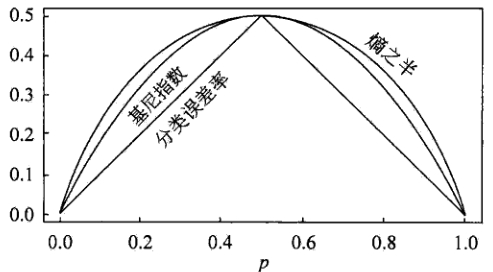其中，横坐标表示概率$p$，纵坐标表示损失。可以看出基尼指数和熵的一半的曲线很接近，都可以近似地代表分类误差率。
分类树的剪枝
剪枝（pruning）是决策树学习算法对付“过拟合”的主要手段。在决策树学习中，为了尽可能正确分类训练样本，结点划分过程将不断重复，有时会造成决策树分支过多，这时就可能因为对训练样本学习得“太好”了，以致于把训练集自身的一些特点当作所有数据都具有的一般性质而导致过拟合。因此，可通过主动去掉一些分支来降低过拟合的风险。决策树剪枝的基本策略有预剪枝和后剪枝。
预剪枝
预剪枝是指在决策树生成过程中，对每个结点在划分前先进行估计，若当前结点的划分不能带来决策树泛化性能提升，则停止划分并将当前结点标记为叶结点。停止决策树生长常用方法：

定义一个高度，当决策树达到该高度时就停止决策树的生长
达到某个节点的实例具有相同的特征向量，即使这些实例不属于同一类，也可以停止决策树的生长。这个方法对于处理数据的数据冲突问题比较有效。
定义一个阈值，当达到某个节点的实例个数小于阈值时就可以停止决策树的生长
定义一个阈值，通过计算每次扩张对系统性能的增益，并比较增益值与该阈值大小来决定是否停止决策树的生长。

后剪枝
后剪枝则是先从训练集生成一棵完整的决策树，然后自底向上地对非叶结点进行考察，若将该结点对应的子树替换为叶结点能带来决策树泛化性能提升，则将该子树替换为叶结点。相比于预剪枝，后剪枝更常用，因为在预剪枝中精确地估计何时停止树增长很困难。
错误率降低剪枝（REP，Reduced-Error Pruning）
错误率降低剪枝方法是一种比较简单的后剪枝的方法。在该方法中，可用的数据被分成两个样例集合：首先是训练集，它被用来形成学习到的决策树，另一个是与训练集分离的验证集，它被用来评估这个决策树在后续数据上的精度，确切地说是用来评估修剪这个决策树的影响。学习器可能会被训练集中的随机错误和巧合规律所误导，但验证集合不大可能表现出同样的随机波动。所以验证集可以用来对过度拟合训练集中的虚假特征提供防护检验。
错误率降低剪枝方法考将树上的每个节点作为修剪的候选对象，再决定是对该节点进行剪枝：

删除以此结点为根的子树
使其成为叶子结点
当修剪后的树对于验证集合的性能不比修剪前的树的性能差时，则确认删除该结点，否则恢复该节点

因为训练集合的过拟合，使得验证集合数据能够对其进行修正，反复进行上面的操作，从底向上的处理结点，删除那些能够提高验证集合的精度的结点，直到进一步修剪会降低验证集合的精度为止。
错误率降低剪枝方法是最简单的后剪枝方法之一，不过由于使用独立的测试集，原始决策树相比，修改后的决策树可能偏向于过度修剪。这是因为一些不会再次在测试集中出现的很稀少的训练集实例所对应的分枝在剪枝过程中往往会被剪枝。尽管错误率降低剪枝方法有这个缺点，不过错误率降低剪枝方法仍然作为一种基准来评价其它剪枝算法的性能。它对于两阶段决策树学习方法的优点和缺点提供了一个很好的学习思路。由于验证集合没有参与决策树的构建，所以用错误率降低剪枝方法剪枝后的决策树对于测试样例的偏差要好很多，能够解决一定程度的过拟合问题。
悲观错误剪枝（PEP，Pesimistic-Error Pruning）
悲观错误剪枝方法是根据剪枝前后的错误率来判定子树的修剪。它不需要像错误率降低修剪方法那样，需要使用部分样本作为测试数据，而是完全使用训练数据来生成决策树，并进行剪枝，即决策树生成和剪枝都使用训练集。
该方法引入了统计学中连续修正的概念弥补错误率降低剪枝方法中的缺陷，在评价子树的训练错误公式中添加了一个常数，即假定每个叶子结点都自动对实例的某个部分进行错误的分类。
把一颗具有多个叶子节点的子树的分类用一个叶子节点来替代的话，在训练集上的误判率肯定是上升的，但是在新数据上不一定。于是我们把子树的误判计算加上一个经验性的惩罚因子来做是否剪枝的考量指标。对于一个叶子节点，它覆盖了$N$个样本，其中有$E$个错误，那么该叶子节点的错误率为$\frac{E + 0.5}{N}$。这个$0.5$就是惩罚因子，那么一颗子树，它有$L$个叶子节点，那么该子树的误判率估计为：
$\frac{\sum E_i +0.5 * L}{\sum N_i}$
这样的话，我们可以看到一棵子树虽然具有多个子节点，但由于加上了惩罚因子，所以子树的误判率计算未必占到便宜。剪枝后内部节点变成了叶子节点，其误判个数$E$也需要加上一个惩罚因子，变成$E+0.5$，那么子树是否可以被剪枝就取决于剪枝后的错误$E+0.5$在的标准误差内。对于样本的误差率$e$，我们可以根据经验把它估计成各种各样的分布模型，比如二项式分布、正态分布等。如果$E+0.5 < E_i + SE(E_i)$则对$i$进行剪枝。
代价复杂度剪枝（CCP，Cost-Complexity Pruning)
代价复杂度剪枝算法为子树$T_t$定义了代价和复杂度，以及一个可由用户设置的衡量代价与复杂度之间关系的参数$α$。其中，代价指在剪枝过程中因子树$T_t$被叶节点替代而增加的错分样本，复杂度表示剪枝后子树$T_t$减少的叶结点数，$α$则表示剪枝后树的复杂度降低程度与代价间的关系，定义为：
$\alpha = \frac{R(t) - R(T_t)}{|N_t| - 1}$
其中，$|N_t|$是子树$T_t$中的叶节点数，$R(t) = r(t) * p(t)$为结点$t$的错误代价，$r(t)$为结点$t$的错分样本率，$p(t)$为落入结点$t$的样本占所有样本的比例，$R(T_t) = \sum R(i)$是子树$T_t$错误代价，$i$为子树$T_t$的叶节点。

对于完全决策树$T$的每个非叶结点计算$α$值，循环剪掉具有最小$α$值的子树，直到剩下根节点，得到一系列的剪枝树$\{ T_0, T_1, T_2, \cdots, T_m \}$，其中$T_0$为原有的完全决策树，$T_m$为根结点，$T_{i +1}$为对$T_i$进行剪枝的结果
从子树序列中，根据真实的误差估计选择最佳决策树

REP
PEP
CCP

剪枝方式
自底向上
自顶向下
自底向上

计算复杂度
$O(n)$
$O(n)$
$O(n^2)$

误差估计
测试集上误差估计
使用连续纠正
标准误差

回归树
建立回归树的过程大致可以分为两步：

将预测变量空间（$X_1, X_2, X_3, \cdots, X_p$）的可能取值构成的集合分割成$J$个互不重叠的区域$\{ R_1, R_2, R_3, \cdots, R_J\}$
对落入区域$R_j$的每个观测值作同样的预测，预测值等于$R_j$上训练集的各个样本取值的算术平均数。

比如，在第一步中得到两个区域$R_1$和$R_2$，$R_1$中训练集的各个样本取值的算术平均数为10，$R_2$中训练集的各个样本取值的算术平均数为20。则对给定的观测值$X = x$，若$x \in R_1$，给出的预测值为10，若$x \in R_2$，则预测值为20。
所以，类似于上述决策树分类算法的第(10)步，关键在于如何构建区域划分$\{ R_1, R_2, R_3, \cdots, R_J\}$。事实上，区域的形状是可以为任意形状的，但出于模型简化和增强可解释性的考虑，这里将预测变量空间划分成高维矩形，我们称这些区域为称盒子。划分区域的目标是找到使模型的残差平方和$RSS$最小的矩形区域$\{ R_1, R_2, R_3, \cdots, R_J\}$。$RSS$的定义为：
$RSS = \sum^J_{j=1} \sum_{i \in R_j}(y_i - \hat{y}_{R_j})^2$
其中，$\hat{y}_{R_j}$是第$j$个矩形区域中训练集中各个样本取值的算术平均数。但是，要想考虑将特征空间划分为$J$个矩形区域的所有可能性，在计算上是不可行的。因此一般采用一种自上而下的贪婪法：递归二又分裂。“自上而下”指的是它从树顶端开始依次分裂预测变量空间，每个分裂点都产生两个新的分支。“贪婪”意指在建立树的每一步中，最优分裂确定仅限于某一步进程，而不是针对全局去选择那些能够在未来进程中构建出更好的树的分裂点。
在执行递归二又分裂时，先选择预测变量$X_j$和分割点$s$，将预测变量空间分为两个区域$\{ X|X_j 和$\{ X|X_j \geq s \}$，使$RSS$尽可能地减小。也就是说，考虑所有预测变量$X_1, X_2, X_3, \cdots, X_p$和与每个预测变量对应的$s$的取值，然后选择预测变量和分割点，使构造出的树具有最小的$RSS$。更详细地，对$j$和$s$，定义一对半平面：
$R_1(j, s) = \{ X|X_j
寻找$j$和$s$，使得下式最小：
$\sum_{x_i \in R_1(j, s)}(y_i - \hat{y}_{R_1})^2 + \sum_{x_i \in R_2(j, s)}(y_i - \hat{y}_{R_2})^2$
重复上述步骤，寻找继续分割数据集的最优预测变量和最优分割点，使随之产生的区域中的$RSS$达到最小。此时被分割的不再是整个预测变量空间，而是之前确定的两个区域之一。如此一来就能得到3个区域。接着进一步分割3个区域之一以最小化$RSS$。这一过程不断持续，直到符合某个停止准则，如我们在分类决策树中讨论到的前剪枝中的停止准则。
区域$\{ R_1, R_2, R_3, \cdots, R_J\}$产生后，就可以确定某一给定的测试数据所属的区域，并用这一区域训练集的各个样本取值的算术平均数作为与测试进行预测。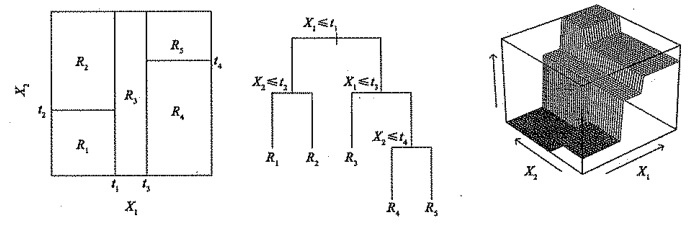回归树的剪枝
上述方法生成的回归树会在训练集中取得良好的预测效果，却很有可能造成数据的过拟合，导致在测试集上效果不佳。原因在于这种方法产生的树可能过于复杂。一棵分裂点更少、规模更小（区域$\{ R_1, R_2, R_3, \cdots, R_J\}$的个数更少）的树会有更小的方差和更好的可解释性（以增加微小偏差为代价）。针对上述问题，一种可能的解决办法是：仅当分裂使残差平方和$RSS$的减小量超过某阈值时，才分裂树结点。这种策略能生成较小的树，但可能产生过于短视的问题，一些起初看来不值得的分裂却可能之后产生非常好的分裂。也就是说在下一步中，$RSS$会大幅减小。
因此，更好的策略是生成一棵很大的树$T_0$，然后通过后剪枝得到子树。直观上看，剪枝的目的是选出使测试集预测误差最小的子树。子树的测试误差可以通过交叉验证或验证集来估计。但由于可能的子树数量极其庞大，对每一棵子树都用交叉验证来估计误差太过复杂。因此需要从所有可能的子树中选出一小部分再进行考虑。在回归树中，我们一般使用代价复杂度剪枝（CCP，Cost-Complexity Pruning），也称最弱联系剪枝（Weakest Link Pruning）。这种方法不是考虑每一棵可能的子树，而是考虑以非负调整参数$\alpha$标记的一系列子树。每一个$\alpha$的取值对应一棵子树$T \in T_0$，当$\alpha$一定时，其对应的子树使下式最小：
$\sum_{m=1}^{|T|} \sum_{x_i \in R_m}(y_i - \hat{y}_{R_m})^2 + \alpha |T|$
这里的$|T|$表示树$T$的结点数，$R_m$是第$m$个终端结点对应的矩形（预测向量空间的一个子集），$\hat{y}_{R_m}$是与$R_m$对应的预测值，也就是$R_m$中训练集的平均值。调整系数$\alpha$在子树的复杂性和与训练数据的契合度之间控制权衡。当$\alpha = 0$时，子树$T$等于原树$T_0$，因为此时上式只衡量了训练误差。而当$\alpha$增大时，终端结点数多的树将为它的复杂付出代价，所以使上式取到最小值的子树会变得更小。
当$\alpha$从0开始逐渐增加时，树枝以一种嵌套的、可预测的模式被修剪，因此获得与$\alpha$对应的所有子树序列是很容易的。可以用交又验证或验证集确定$\alpha$，然后在整个数据集中找到与之对应的子树：

回归决策树算法
1.利用递归二叉分裂在训练集中生成一额大树，只有当终端结点包含的观测值个数低于某个最小值时才停止。
2.对大树进行代价复杂性剪枝，得到一系列最优子树，子树是$\alpha$的函数。
3.利用$K$折交叉验诞选择$\alpha$。具体做法是将训练集分为$K$折。对所有$k=1, 2, 3, \cdots, K$，对训练集上所有不属于第$k$折的数据重复第(1)步~第(2)步得到与$\alpha$对应的子树，并求出上述子树在第$k$折上的均方预测误差。
4.每个$\alpha$会有相应的$K$个均方预测误差，对这$K$个值求平均，选出使平均误差最小的$\alpha$。
5.找出选定的$\alpha$在第(2)步中对应的子树。

决策树的基础即是上文所述的分类决策树与回归决策树，其预测准确性一般比回归和分类方法弱，但可以通过用集成学习方法组合大量决策树，显著提升树的预测效果，这些方法我将会在接下来的文章中继续阐述，欢迎关注学习讨论！


展开全文cart分类回归树 回归树
• 决策树 决策树(Decision Tree）是在已知各种情况发生概率的基础上，通过构成...在机器学习中，决策树是一个预测模型，他代表的是对象属性与对象值之间的一种映射关系。Entropy = 系统的凌乱程度，使用算法ID3, C4....
决策树
决策树(Decision Tree）是在已知各种情况发生概率的基础上，通过构成决策树来求取净现值的期望值大于等于零的概率，评价项目风险，判断其可行性的决策分析方法，是直观运用概率分析的一种图解法。由于这种决策分支画成图形很像一棵树的枝干，故称决策树。在机器学习中，决策树是一个预测模型，他代表的是对象属性与对象值之间的一种映射关系。Entropy = 系统的凌乱程度，使用算法ID3, C4.5和C5.0生成树算法使用熵。这一度量是基于信息学理论中熵的概念。
决策树是一种树形结构，其中每个内部节点表示一个属性上的测试，每个分支代表一个测试输出，每个叶节点代表一种类别。
分类树（决策树）是一种十分常用的分类方法。他是一种监管学习，所谓监管学习就是给定一堆样本，每个样本都有一组属性和一个类别，这些类别是事先确定的，那么通过学习得到一个分类器，这个分类器能够对新出现的对象给出正确的分类。这样的机器学习就被称之为监督学习。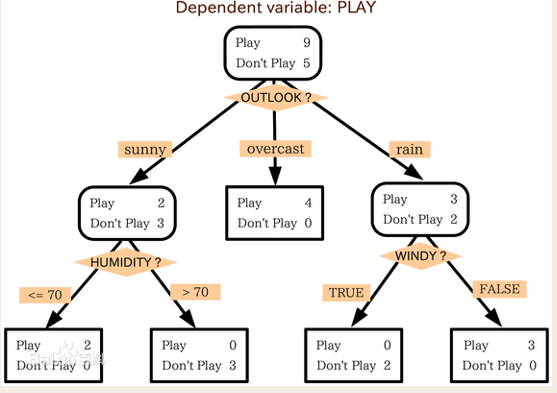决策树可应用于银行信用自动评估系统
1 决策树的优点：
直观，便于理解，小规模数据集有效
2 决策树的缺点：
处理连续变量不好
类别较多时，错误增加的比较快
规模性一般
3 创建决策树
3.1熵
比特（bit)来衡量信息的多少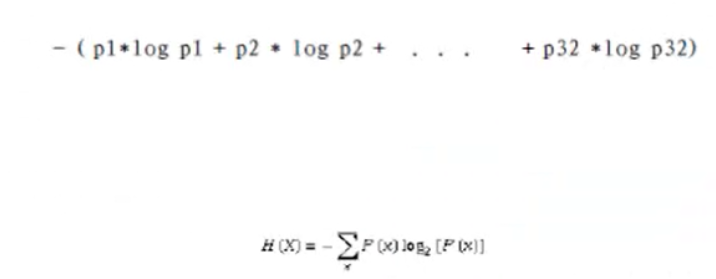变量的不确定性越大，熵也就越大。
3.2信息增益
信息增益（Information Gain)：Gain(A)=Info(D)-Infor_A(D)
通过A来作为节点分类获取了多少信息。
示例：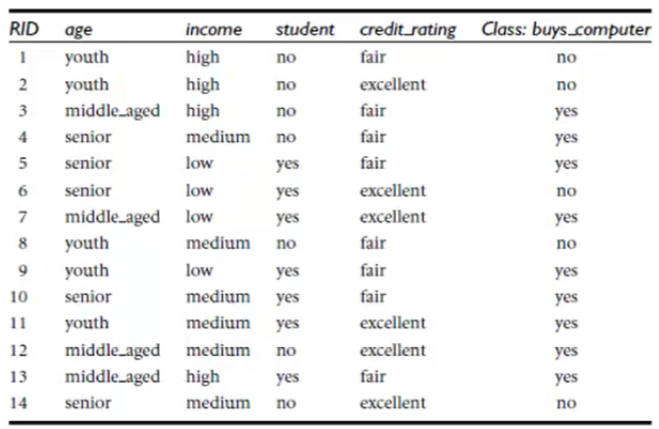总的信息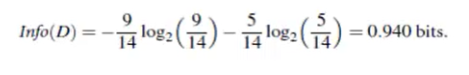以年龄为节点的信息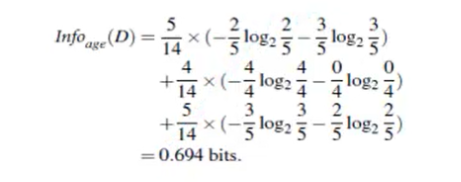信息增益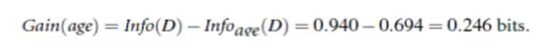类似的，计算出Gain(income)=0.029,Gain(student)=0.151,Gain(credit_rating)=0.048
所以，由于age的信息增益最大，选择age作为第一个根节点。
4  python代码实现
4.1创建csv文件

打开excel文件，输入表格数据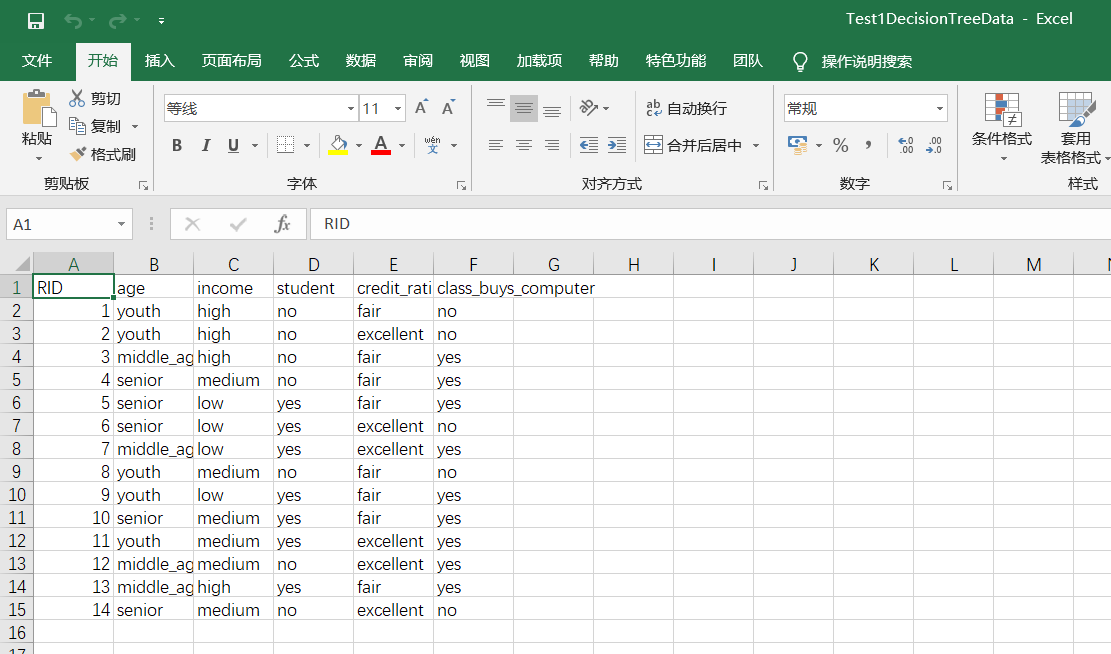点击另存为，csv（逗号隔开）格式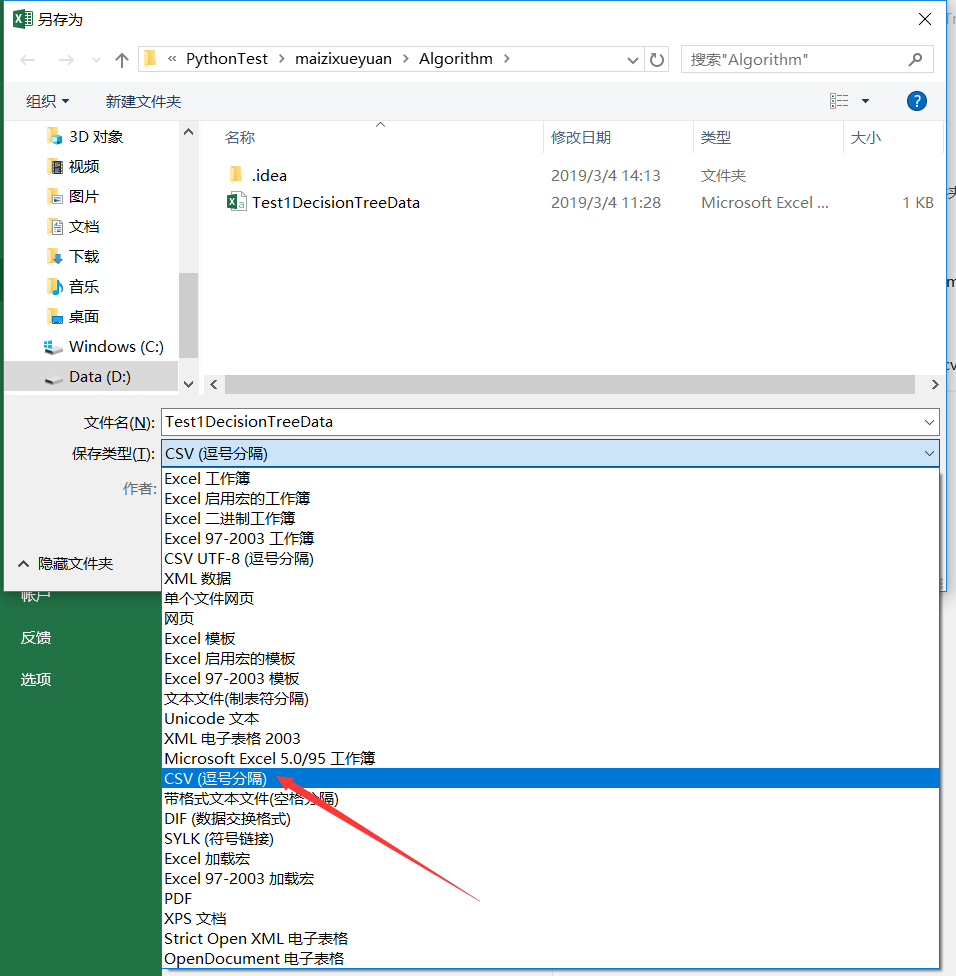4.2 python代码
# -*- coding:utf-8 -*-
#决策时的应用算法
"""
scikit-learn 强大的机器学习库 达到商用级别
对数据输入的要求
对所有的特征值必须是数值型
"""
# print("hello world")
from sklearn.feature_extraction import DictVectorizer
import csv
from sklearn import preprocessing #需要使用到预处理
from sklearn import tree #需要使用到树
# from sklearn.externals.six import StringIO #读写功能

Test1DecisionTreeData = open(r'D:\PythonTest\maizixueyuan\Algorithm\Test1DecisionTreeData.csv','rt')  #读取表格数据

feature_list = []
label_list = []

label_list.append(row[len(row)-1])
#print(row)
row_dict = {}
for i in range(1, len(row)-1):
feature_list.append(row_dict)

#print(feature_list)

vec = DictVectorizer()
dummyX = vec.fit_transform(feature_list).toarray()
print("dummyX:\n" + str (dummyX)) #在特征值上将字符型的转化为数值
print(vec.get_feature_names())

print("label_list:" + str(label_list))

lb = preprocessing.LabelBinarizer() #在标签上进行二进制化
dummyY = lb.fit_transform(label_list)
print("dummyY:\n" + str(dummyY))

clf = tree.DecisionTreeClassifier(criterion = 'entropy')
clf = clf.fit(dummyX,dummyY)
print("clf=:"+str(clf))

with open("tree.dot",'w') as f:
f = tree.export_graphviz(clf, feature_names=vec.get_feature_names(), out_file= f)

OneRowX = dummyX[0,:]
print("OneRowX:\n"+str(OneRowX))

newRowX = OneRowX

newRowX = 1
newRowX = 0
print("NewRowX:\n"+str(newRowX))  #更改后需要预测的数据

predictedY = clf.predict([newRowX])  #预测，记住clf.predict()内加[]
print("predictedY:"+str(predictedY))  #预测值1为能买电脑

读取表格数据时，改为你的csv存放路径
运行成功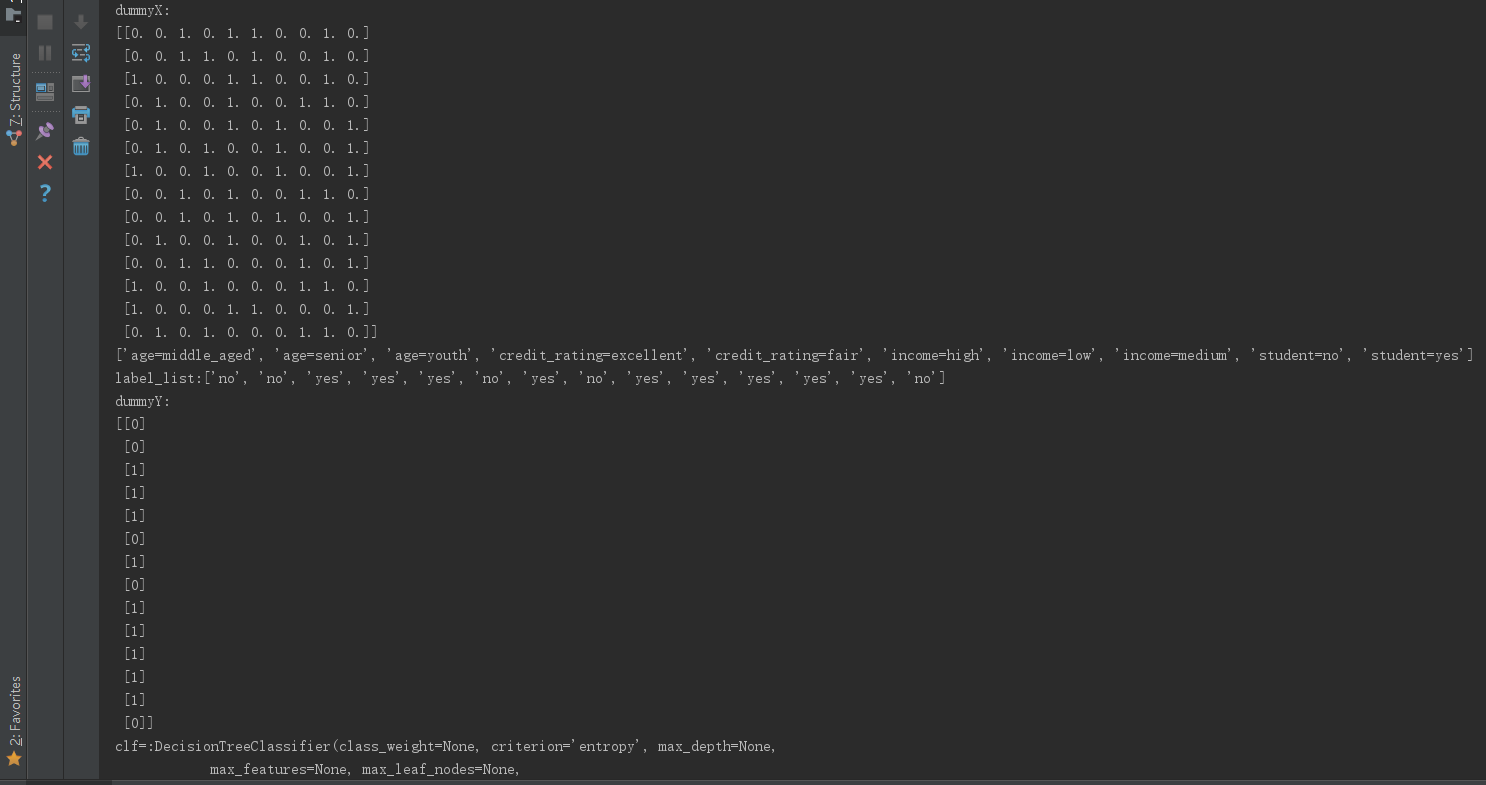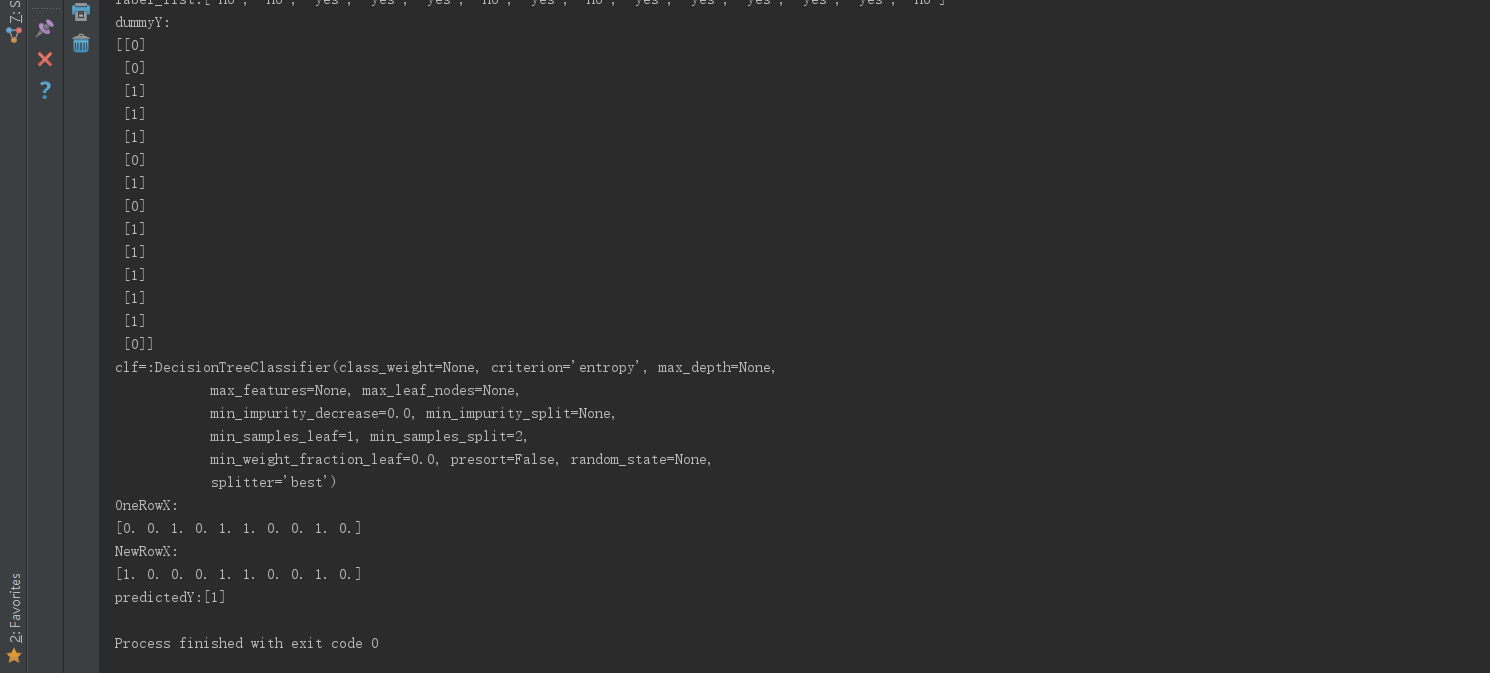运行后打开代码文档路径，会发现生成了一个tree.dot文件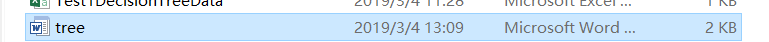打开文档为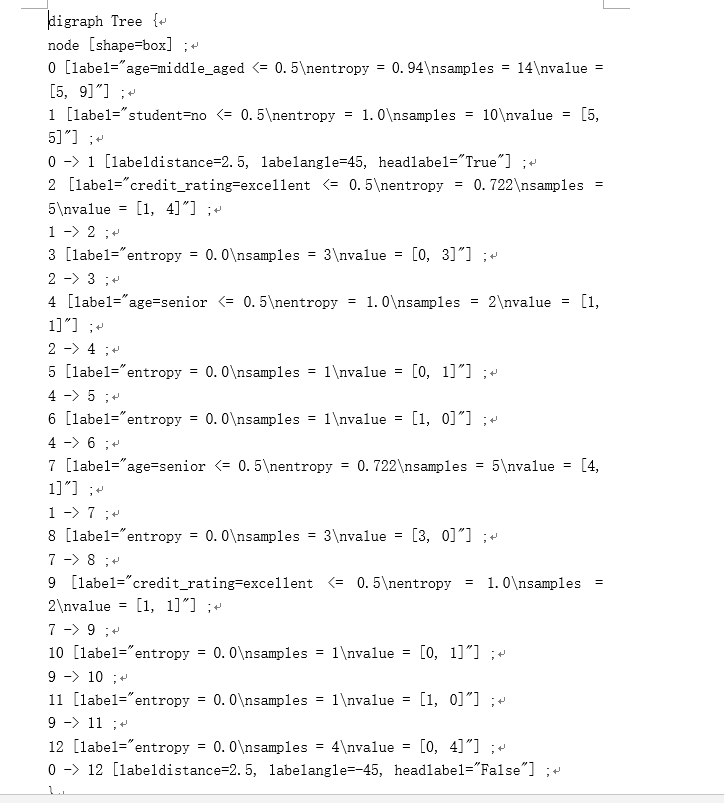5 将dot转换为可视化PDF或PNG
5.1 Windows下载及安装 Graphviz

双击graphviz-2.38.msi下载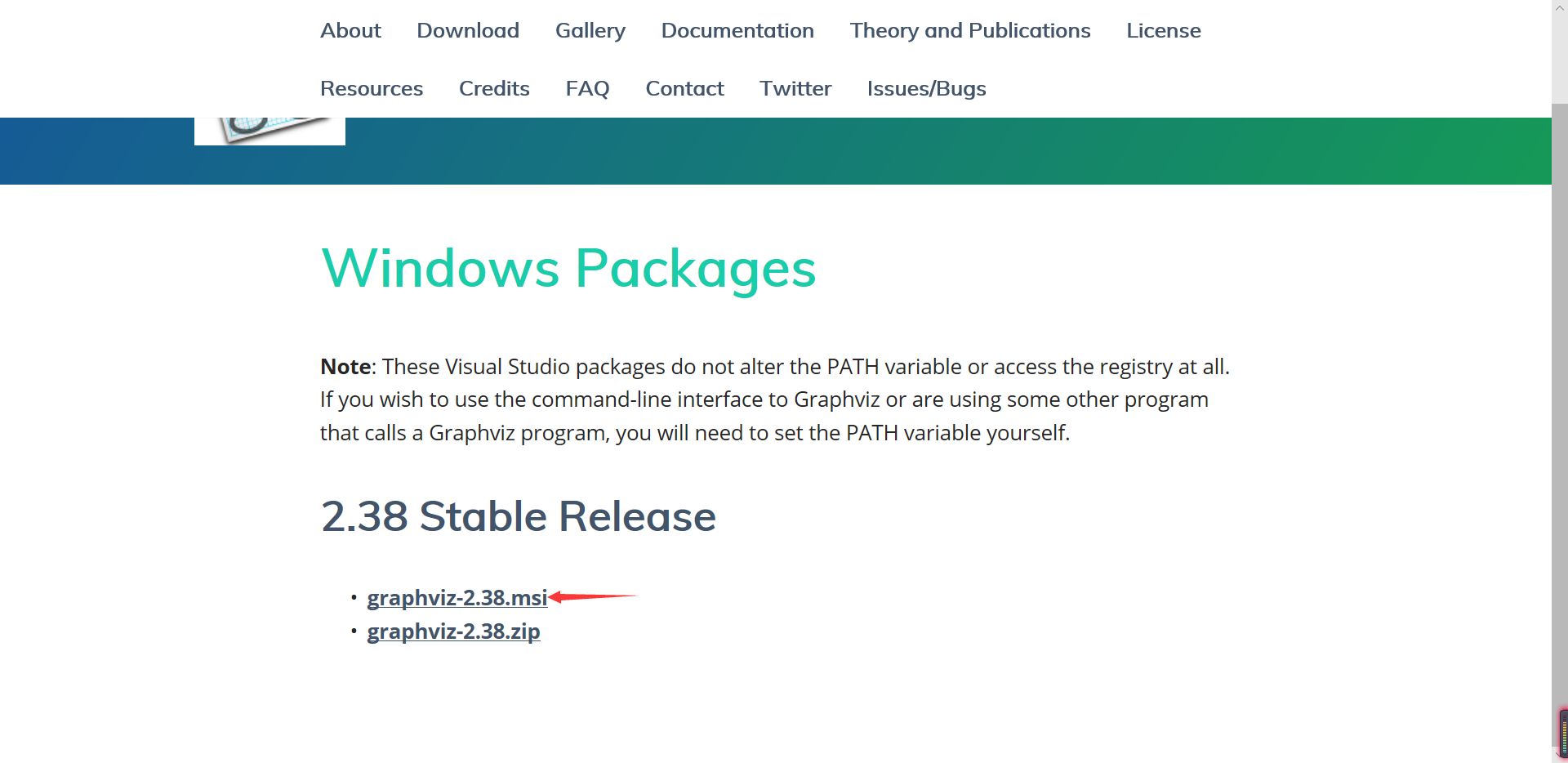下载，安装，记住安装路径（或最好自己安装新建到自己熟悉的能找到的路径下）
安装好后，在命令行里搜索环境变量，点击高级下的环境变量找到path，双击进去，新建，将D:\Software\Graphviz\bin  直到bin的路径添加进去，然后确定就配置好了环境。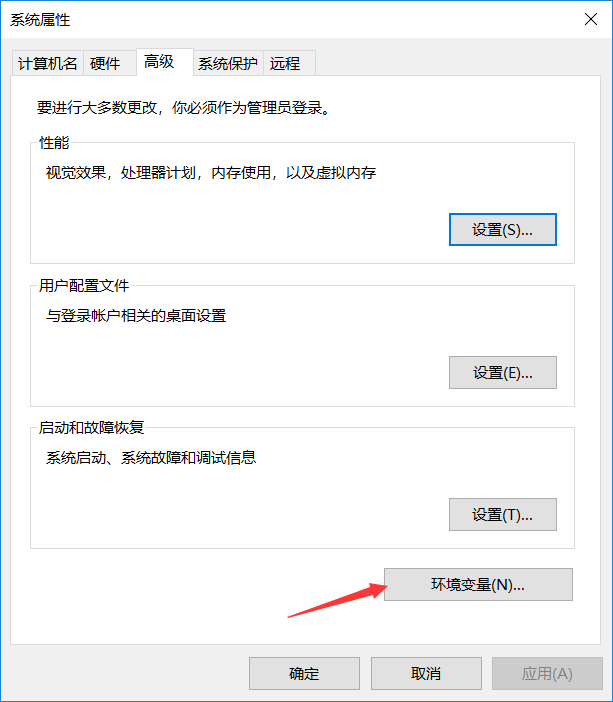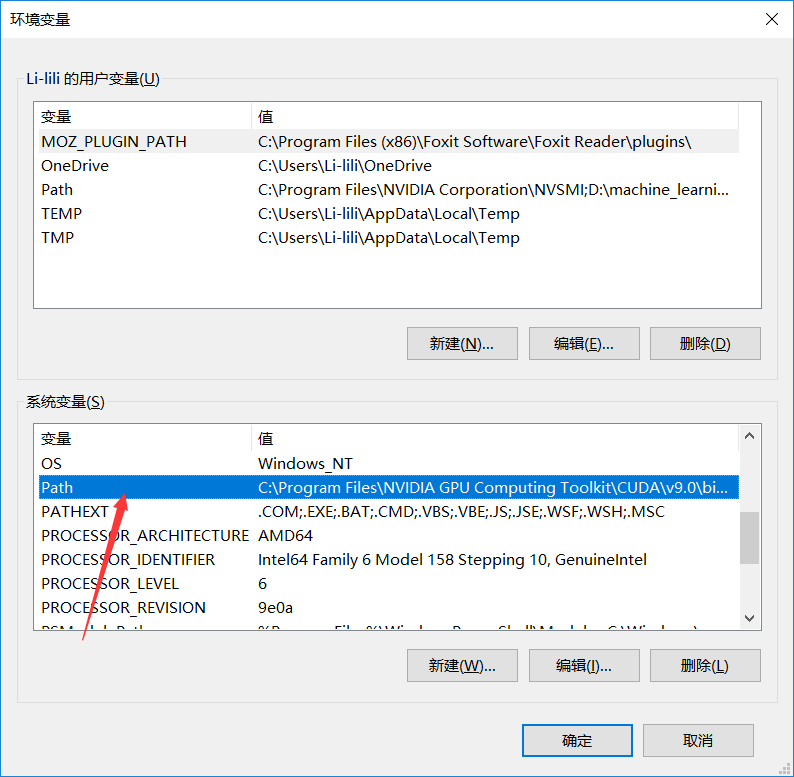测试是否安装好，打开cmd命令管理界，输入dot -vision,出现以下图片所示，则为安装成功。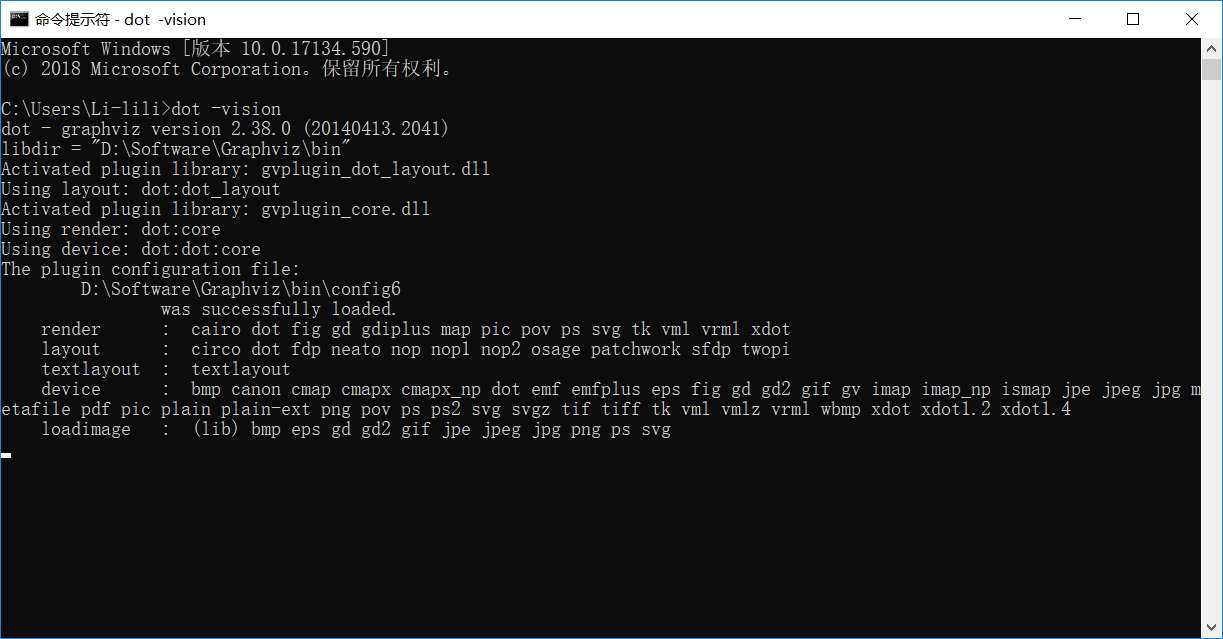5.2 将决策树dot转换成pdf

找到生成tree.dot文件路径，复制路径，在cmd中进入该路径下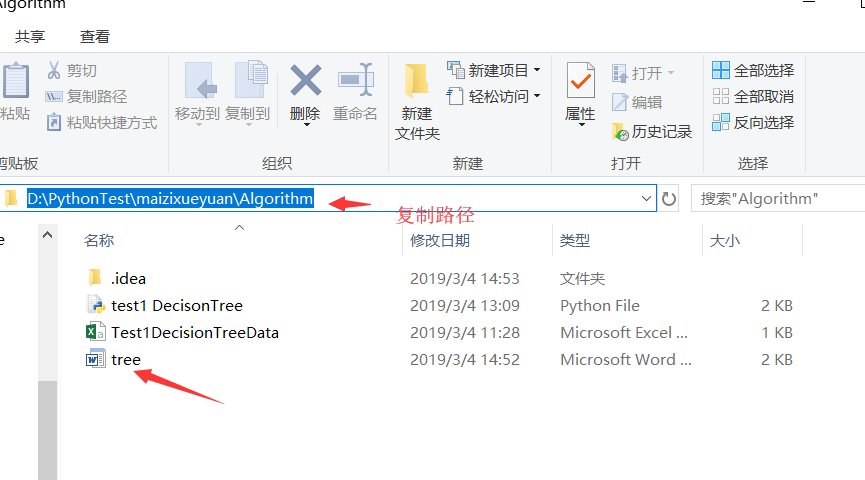cmd到该文件路径下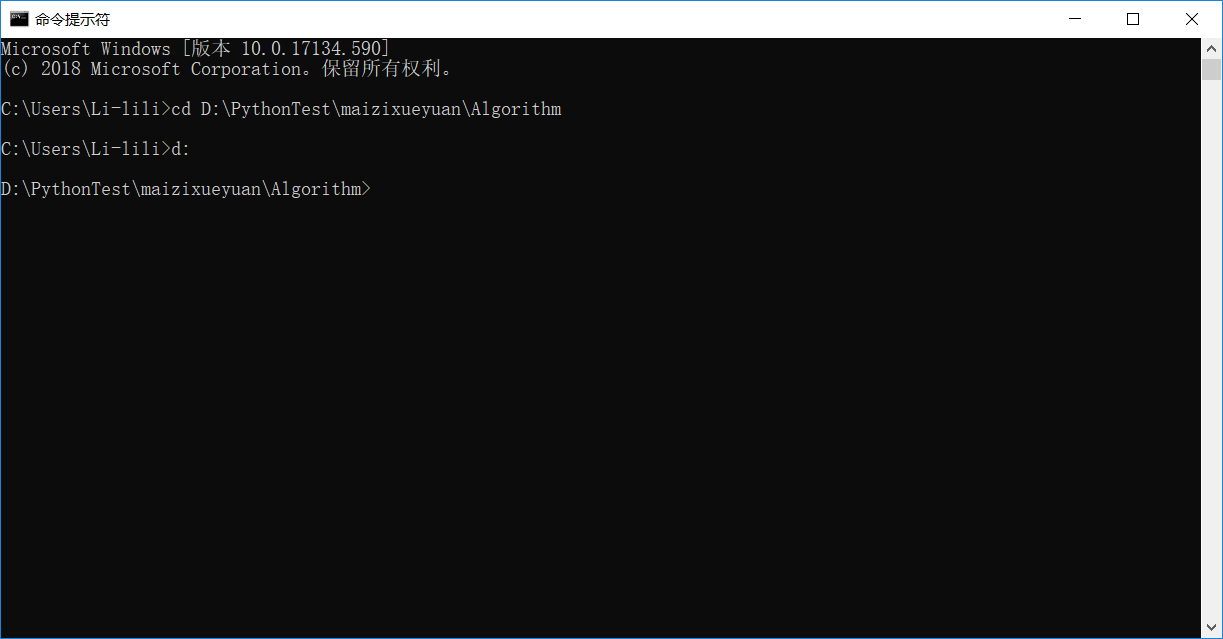输入dot -Tpdf tree.dot -o tree.pdf,出现如下则成功转换。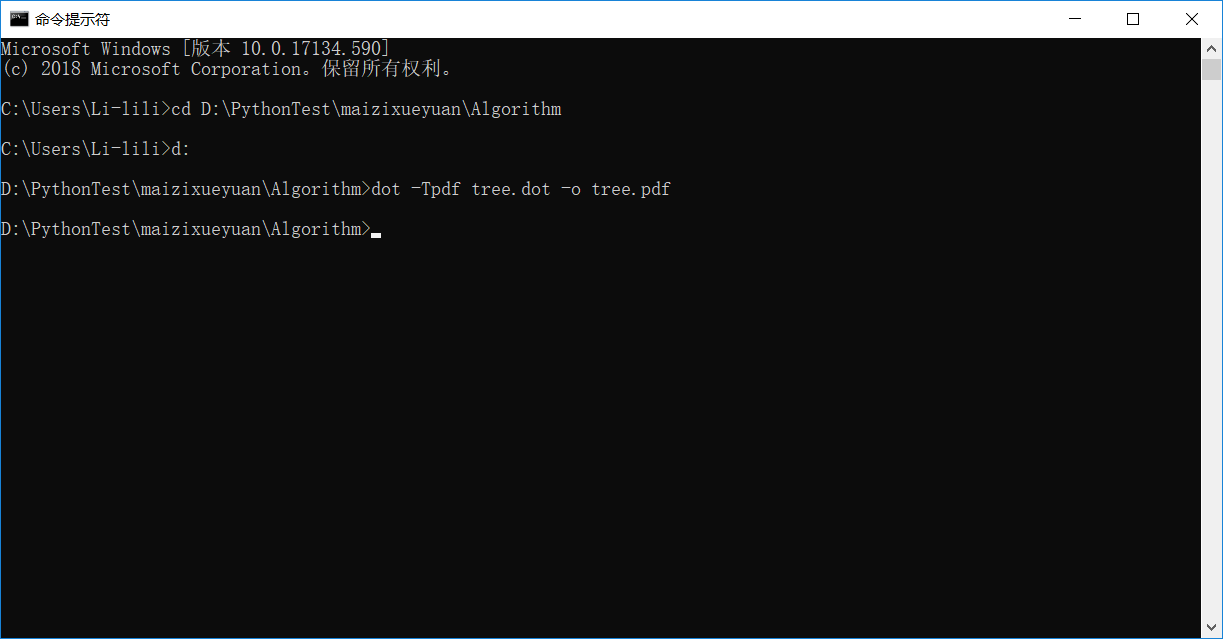4.返回tree.dot文件目录下，会发现生成了一个tree.pdf文件，打开如下则完成。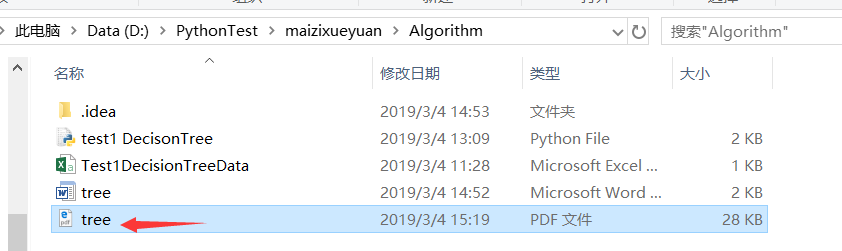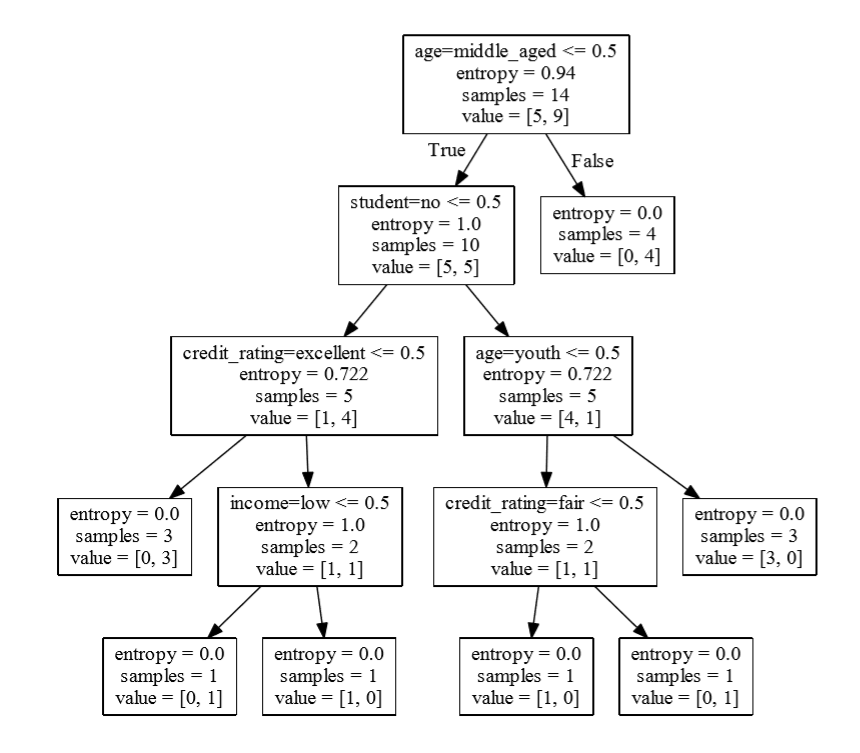`
展开全文Graphviz
• 1. 决策树的优势决策树作为处理分类问题最经常被用到的算法，它有着优于其他机器学习算法的简明性特征，即无需了解机器学习的原理知识也能够轻松的了解这个算法是如何工作的。2.决策树的构造 (1). 找到决定性的特征...算法
• #从文档中读取数据，每条数据转成列表的形式 def readData(path): dataList = [] with open(path,'r') as f: dataSet = f.readlines() for d in dataSet: d = d[:-1] d = d.split(',') dataList.append(d) ...python
• 工具：PythonCharm 书中的代码是python2的，而我用的python3，结合实践过程，这里会标注实践时遇到的问题和针对python3的修改。  实践代码和训练测试数据可以参考这里  ...【决策树算法 ID3】  首先附实例
• 首先我们要了解python机器学习决策树用来做什么的，然后清楚的知道算法原理，最后才能将决策树算法应用到实际场景当中。 决策树，就是一种把决策节点画成树的辅助决策工具，一种寻找最优方案的画图法。画决策树有...
• 本文为本人在学习机器学习决策树部分的一些笔记和思考，以及python编程实现算法的具体步骤 决策树(decision tree) 是一类常见的机器学习方法. 在已知各种情况发生概率的基础上，通过构成决策树来求取净现值的期望值...
• 机器学习决策树，讲解ID3、CART、C4.5树，涉及信息增益，增益率，基尼指数，剪枝策略，多属性结合
• 有读者反映，说我上篇文章Python3《机器学习实战》学习笔记（一）：k-近邻算法(史诗级干货长文)，太长了。一看那么长，读的欲望都降低了。既然如此，决策树的内容，我就分开讲好了。本篇讨论决策树的原理和决策树的...
• 机器学习算法与Python实践(11) - 决策树 ID3、C4.5、CART目录　什么是决策树(Decision Tree) 　特征选择 　使用ID3算法生成决策树 　使用C4.5算法生成决策树 　使用CART算法生成决策树 　预剪枝和后剪枝 　...算法
• 本文数据参照 机器学习-周志华 一书中的决策树一章。可作为此章课后习题4的答案 代码则参照《机器学习实战》一书的内容，并做了一些修改。 CART决策树 使用基尼指数（Gini Index）来选择划分属性。其公式如下： ...
•实战
• 1.背景  接着上一节说，没看到请先看一下上一节关于数据集的划分数据集划分。现在我们得到了每个特征值得信息熵增益，我们按照信息熵增益的从大到校的...（二叉树的图是用python的matplotlib库画出来的） 数据集：
• 下面我们就来看看sklearn是如何来实现决策树中的分类树的 本文目录： 1 概述 1.1sklearn中的决策树 2 DecisionTreeClassififier与红酒数据集 2.1 重要参数 2.1.1 criterion ...决策树
• 本文数据参照 机器学习-周志华 一书中的决策树一章。可作为此章课后习题3的答案 代码则参照《机器学习实战》一书的内容，并做了一些修改。 本文使用的Python库包括 numpypandasmathoperatormatplotlib 本文...
• 在看了决策树相关内容和麦子学院讲解的利用Python构建决策树的视频教程后，跟着视频做了构建决策树的过程 本次构建决策树的使用的算法是ID3算法，主要思想是利用不同特征值的信息熵来作为最优划分属性，决策树具体...
• 根据所学知识，可采用sklearn中的决策树等方法进行程序设计。欢迎大家一起讨论学习进步。 训练集和测试集链接如下：   一. 设计思路 1.读取训练集和测试集文件 2.对数据进行处理 3.训练决策树 4.输出预测...sklearn 随机森林
•ID3
• 决策树是一个预测模型；他代表的是对象属性与对象值之间的一种映射关系。树中每个节点表示某个对象，而每个分叉路径则代表的某个可能的属性值，而每个叶结点则对应从根节点到该叶节点所经历的路径所表示的对象的值。...数据挖掘 大数据
• 决策树学习通常包含三个步骤：特征选择，决策树的生成，决策树的剪枝。 优点：计算复杂度不高，输出结果易于理解，对中间值的缺失值不敏感，可以处理不相关特征数据。 缺点：可能会产生过度匹配的问题。 使用...
• ">我们知道建立一个决策树是很好时间的，我们在一个大的数据集下建立的决策树费时很久，我们希望下次可以直接用而不是再生成一遍，所以我们要存储已建好的决策树。 ;">;">def storeTree(inputTree,filename): ...matplotlib 分类器...

# 机器学习决策树pythonpython 订阅